• 2021-04-29 16:31:40

本来以为这个人写的挺好，但是发现似乎有些问题，而且比较繁琐。这个人也是。
https://www.cnblogs.com/empty16/p/6222885.html
https://www.cnblogs.com/coolqiyu/p/5580290.html
后来发现这个人写还算不错，至少能够用起来，没有报错，但里面有些用法感觉查了资料才会理解。
https://www.cnblogs.com/tsingke/p/5833221.html
https://www.pianshen.com/article/4718305185/

LaTeX 写作： 算法代码排版 --latex2e范例总结

latex2e 宏包的使用范例：

• \usepackage[ruled]{algorithm2e}                                     %算法排版样式1
• \usepackage[ruled,vlined]{algorithm2e}                          %算法排版样式2
• \usepackage[linesnumbered,boxed]{algorithm2e}        %算法排版样式3

使用上述代码中三种不同的宏包编译后的代码排版样式预览：

样式一：样式二：样式三：latex代码排版.tex文件：

\documentclass{article}

\usepackage[ruled]{algorithm2e}                 %算法排版样式1
%\usepackage[ruled,vlined]{algorithm2e}          %算法排版样式2
%\usepackage[linesnumbered,boxed]{algorithm2e}   %算法排版样式3

\begin{document}

How to use the algorithm2e in \LaTeX ~ file.

Examples:

%    ------------------------------Example - 1---------------------------------------------
\begin{algorithm}[H]
\caption{How to write algorithms}
\KwIn{this text}
\KwOut{how to write algorithm with \LaTeX2e }

initialization\;
\While{not at end of this document}{
\eIf{understand}
{
go to next section\;
current section becomes this one\;
}
{
go back to the beginning of current section\;
}
}
\end{algorithm}

%    ---------------------------Example - 2------------------------------------------------
\begin{algorithm}
%    \SetAlgoNoLine  %去掉之前的竖线
\caption{identifyRowContext}
\KwIn{$r_i$, $Backgrd(T_i)$=${T_1,T_2,\ldots ,T_n}$ and similarity threshold $\theta_r$}
\KwOut{$con(r_i)$}
$con(r_i)= \Phi$\;
\For{$j=1;j \le n;j \ne i$}
{
float $maxSim=0$\;
$r^{maxSim}=null$\;
\While{not end of $T_j$}
{
compute Jaro($r_i,r_m$)($r_m\in T_j$)\;
\If{$(Jaro(r_i,r_m) \ge \theta_r)\wedge (Jaro(r_i,r_m)\ge r^{maxSim})$}
{
replace $r^{maxSim}$ with $r_m$\;
}
}
$con(r_i)=con(r_i)\cup {r^{maxSim}}$\;
}
return $con(r_i)$\;
\end{algorithm}
%--------------------------------------------------------------------------------------

some special information
The algorithm2e LaTeX package conflicts with several others over the use of the algorithm identifier.  A common indicator is something like this message:
To resolve the issues, simply put the following just before the inclusion of the algorithm2e package:

%\makeatletter
%\newif\if@restonecol
%\makeatother
%\let\algorithm\relax
%\let\endalgorithm\relax

\end{document}


中文latex模式下，更改Input为输入，更改Output为输出的方法： 在算法内部插入、

\SetKwInOut{KIN}{输入}

\SetKwInOut{KOUT}{输出}


用我们定义的新的宏名“\KIN” 在算法开头相应位置替换掉自带的\KwInput，用"\KOUT" 替换掉\KwOutput 即可，实现中文的输入和输出.

具体事例如下：

1 \renewcommand{\algorithmcfname}{算法}
2 \begin{algorithm}[H]
3 %\SetAlgoNoLine
4 \SetKwInOut{KIN}{输入}
5 \SetKwInOut{KOUT}{输出}
6 %\BlankLine  %空一行
7     \caption{标准DE算法 }
8     \label{DE_algo} %
9     \KIN{Population: $M$; Dimension: $D$; Genetation: $T$ }
10     \KOUT{The best vector (solution)  $\varDelta$ }
11     $t \leftarrow 1 (initialization)$\;
12     \For{$i=1$ to $M$    }
13     {\For{$j=1$ to $D$}
14         {
15             ${x}_{i,t}^j=x_{min}^j + rand(0,1)\cdotp (x_{max}^j-x_{min}^j)$\;
16         }
17     }
18     %--------------------------------------------------
19 \While{$(|f(\varDelta)| \geq\varepsilon )$      or     $(t \leq T )$}
20     {
21         \For{$i=1$  to $M$}
22         {
23 \emph{$\blacktriangleright$ (Mutation and Crossover)}\\
24 %\textit{ $\blacktriangleright$ (Mutation and Crossover) }\\
25             \For{$j=1$ to $D$}
26             {
27                 $v_{i,t}^j =Mutation(x_{i,t}^j)$\;
28                 $u_{i,t}^j =Crossover(x_{i,t}^j,v_{i,t}^j)$\;
29             }
30 \emph{$\blacktriangleright$ (Greedy Selection)}\\
31             %\textit{ $\blacktriangleright$ (Greedy Selection) }\\
32             \eIf{$f(\textbf{u}_{i,t}) < f(\textbf{x}_{i,t})$}
33             {
34                 $\textbf{x}_{i,t} \leftarrow\textbf{u}_{i,t}$\;
35                 \If{$f(\textbf{x}_{i,t}) < f(\varDelta)$}
36                 {
37                     $\varDelta \leftarrow \textbf{x}_{i,t}$ \;
38                 }
39             }
40             {
41                 $\textbf{x}_{i,t} \leftarrow \textbf{x}_{i,t}$\;
42             }
43         }
44         $t \leftarrow t+1$\;
45     }    %While
46     \Return the best vector  $\varDelta$\;
47 \end{algorithm}


### 附加工具：

显示直立文本： \textup{文本}
意大利斜体： \textit{文本}
slanted斜体： \textsl{文本}
显示小体大写文本： 　\textsc{文本}
中等权重： \textmd{文本}
加粗命令： \textbf{文本}
默认值： \textnormal{文本}

斜体字：\textit{italic}，或者 \emph{italic}

细体字：\textlf{light font}

使用等宽字体：\texttt{code}

使用无衬线字体：\textsf{sans-serif}

所有字母大写：\uppercase{CAPITALS}

所有字母大写，但小写字母比较小：\textsc{Small Capitals}


参考：https://www.cnblogs.com/xueqiuqiu/articles/9086713.html
https://blog.csdn.net/u014630987/article/details/70156489

更多相关内容
• ## LaTeX各种算法排版

千次阅读 2020-11-10 16:49:29
%算法的返回值 \end{algorithmic} \end{algorithm} 排版结果如下： 5）一个例子 \begin{algorithm}[h] \caption{An example for format For \& While Loop in Algorithm} \begin{algorithmic} \FOR{each $i \in ... 1）首先在导言区加入语句： \usepackage{algorithm} \usepackage{algorithmic} 2）例1 \begin{algorithm} \caption{A} \label{alg:A} \begin{algorithmic} \STATE {set$r(t)=x(t)$} \REPEAT \STATE set$h(t)=r(t)$\REPEAT \STATE set$h(t)=r(t)$\UNTIL{B} \UNTIL{B} \end{algorithmic} \end{algorithm} 排版结果如下：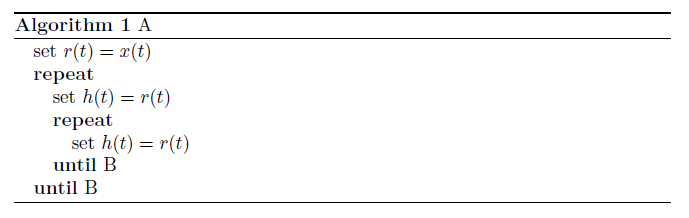3）例2 \begin{algorithm} \caption{Calculate$y = x^n$} \label{alg1} \begin{algorithmic} \REQUIRE$n \geq 0 \vee x \neq 0$\ENSURE$y = x^n$\STATE$y \Leftarrow 1$\IF{$n < 0$} \STATE$X \Leftarrow 1 / x$\STATE$N \Leftarrow -n$\ELSE \STATE$X \Leftarrow x$\STATE$N \Leftarrow n$\ENDIF \WHILE{$N \neq 0$} \IF{$N$is even} \STATE$X \Leftarrow X \times X$\STATE$N \Leftarrow N / 2$\ELSE[$N$is odd] \STATE$y \Leftarrow y \times X$\STATE$N \Leftarrow N - 1$\ENDIF \ENDWHILE \end{algorithmic} \end{algorithm} 排版结果如下：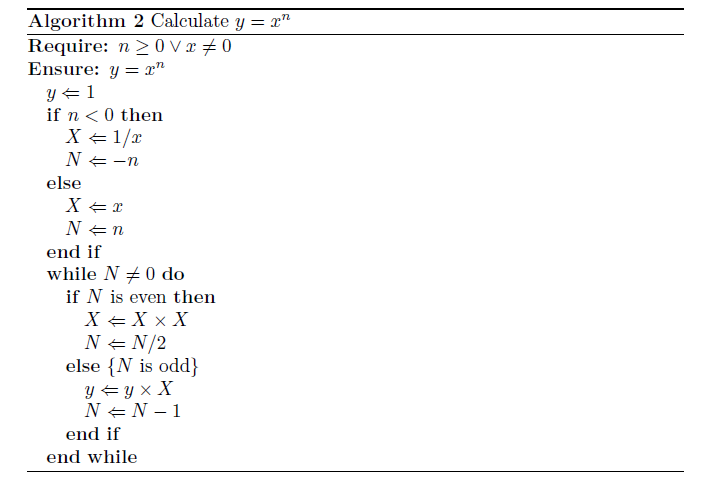4）\renewcommand 改变现有命令的定义。在导言区加入如下语句： \renewcommand{\algorithmicrequire}{ \textbf{Input:}} %Use Input in the format of Algorithm \renewcommand{\algorithmicensure}{ \textbf{Output:}} %UseOutput in the format of Algorithm 使得原来软件包中定义的命令\REQUIRE和\ENSURE显示为Input:和Output: 一个例子如下： \begin{algorithm}[htb] \caption{ Framework of ensemble learning for our system.} \label{alg:Framwork} \begin{algorithmic} %这个1 表示每一行都显示数字 \REQUIRE ~~\\ %算法的输入参数：Input The set of positive samples for current batch,$P_n$;\\ The set of unlabelled samples for current batch,$U_n$;\\ Ensemble of classifiers on former batches,$E_{n-1}$; \ENSURE ~~\\ %算法的输出：Output Ensemble of classifiers on the current batch,$E_n$; \STATE Extracting the set of reliable negative and/or positive samples$T_n$from$U_n$with help of$P_n$; \label{ code:fram:extract }%对此行的标记，方便在文中引用算法的某个步骤 \STATE Training ensemble of classifiers$E$on$T_n \cup P_n$, with help of data in former batches; \label{code:fram:trainbase} \STATE$E_n=E_{n-1}\cup E$; \label{code:fram:add} \STATE Classifying samples in$U_n-T_n$by$E_n$; \label{code:fram:classify} \STATE Deleting some weak classifiers in$E_n$so as to keep the capacity of$E_n$; \label{code:fram:select} \RETURN$E_n$; %算法的返回值 \end{algorithmic} \end{algorithm} 排版结果如下：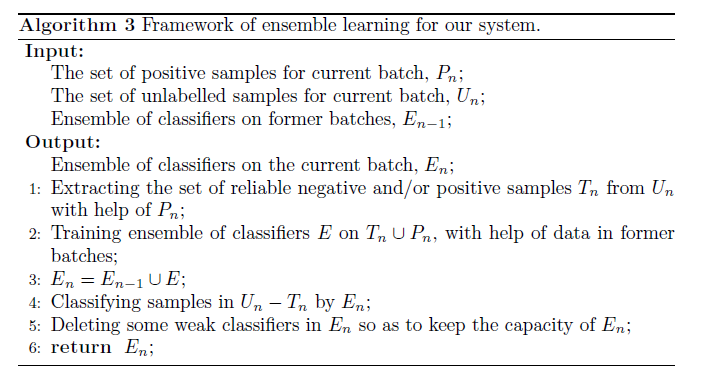5）一个例子 \begin{algorithm}[h] \caption{An example for format For \& While Loop in Algorithm} \begin{algorithmic} \FOR{each$i \in [1,9]$} \STATE initialize a tree$T_{i}$with only a leaf (the root);\ \STATE$T=T \cup T_{i};$\ \ENDFOR \FORALL {$c$such that$c \in RecentMBatch(E_{n-1})$} \label{code:TrainBase:getc} \STATE$T=T \cup PosSample(c)$; \label{code:TrainBase:pos} \ENDFOR \FOR{$i=1$;$i<n$;$i++$} \STATE$//$Your source here; \ENDFOR \FOR{$i=1$to$n$} \STATE$//$Your source here; \ENDFOR \STATE$//$Reusing recent base classifiers. \label{code:recentStart} \WHILE {$(|E_n| \leq L_1 )and( D \neq \phi)$} \STATE Selecting the most recent classifier$c_i$from$D$; \STATE$D=D-c_i$; \STATE$E_n=E_n+c_i$; \ENDWHILE \label{code:recentEnd} \end{algorithmic} \end{algorithm} 排版结果如下：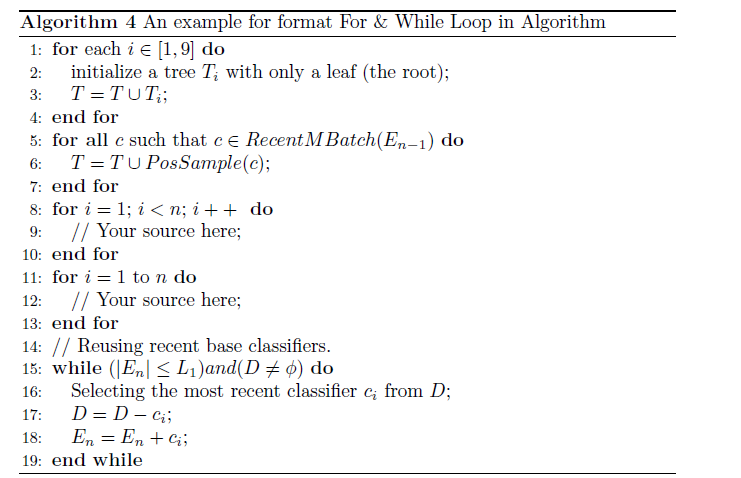6）开始中头部加入 \makeatletter \newenvironment{breakablealgorithm} {% \begin{breakablealgorithm} \begin{center} \refstepcounter{algorithm}% New algorithm \hrule height.8pt depth0pt \kern2pt% \@fs@pre for \@fs@ruled \renewcommand{\caption}[\relax]{% Make a new \caption { \raggedright\textbf{\ALG@name~\thealgorithm} ##2\par}% \ifx\relax##1\relax % #1 is \relax \addcontentsline{loa}{algorithm}{\protect\numberline{\thealgorithm}##2}% \else % #1 is not \relax \addcontentsline{loa}{algorithm}{\protect\numberline{\thealgorithm}##1}% \fi \kern2pt\hrule\kern2pt } }{% \end{breakablealgorithm} \kern2pt\hrule\relax% \@fs@post for \@fs@ruled \end{center} } \makeatother 算法中使用： \begin{breakablealgorithm} \caption{Multi task balanced scheduling algorithm} \label{alg1} \begin{algorithmic} %%%REQUIRE 输入 \REQUIRE ~~\\$G$= 250; Size = 30; CodeL = 10; \FOR{$i = 1:3$} \STATE Min$X(i)$= 0.1 * ones(1); \STATE Max$X(i)$= 3 * ones(1); \ENDFOR \FOR{$i = 4:1:9$} \STATE Min$X(i)$= -3 * ones(1); \STATE Max$X(i)$= 3 * ones(1); \ENDFOR \FOR{$i = 10:1:12$} \STATE Min$X(i)$= -ones(1); \STATE Max$X(i)$= ones(1); \ENDFOR \STATE$E$= round(rand(Size,12*CodeL)); \STATE BsJ = 0; \FOR{$kg = 1:1:G$} \STATE time(kg) = kg \FOR{$s$= 1:1:Size} \STATE$m = E(s,:)$; \FOR{$j = 1:1:12$} \STATE$y(j) = 0;$\STATE$mj = m((j-1)$* CodeL +$1:1:j$* CodeL); \FOR{$i = 1:1:$CodeL} \STATE$y(j) = y(j) + mj(i) * 2^(i - 1);$\ENDFOR \STATE$f(s,j) =$(Max$X(j)$- Min$X(j)$) *$y(j)/1023$+ Min$X(j)$; \ENDFOR \STATE$p = f(s,:);$\STATE [p,BsJ] = fitness(p,BsJ); \STATE BsJi(s) = BsJ; \ENDFOR \STATE [OderJi,IndexJi] = sort(BsJi); \STATE BestJ(kg) = OderJi(1); \STATE BJ = BestJ(kg); \STATE Ji = BsJi + 1e-10; \STATE fi = 1./Ji; \STATE [Oderfi,Indexfi] = sort(fi); \STATE Bestfi = Oderfi(Size); \STATE BestS = E(Indexfi(Size),:); \STATE kg \STATE p \STATE BJ \STATE fi\_sum = sum(fi); \STATE fi\_Size = (Oderfi/fi\_sum) * Size; \STATE fi\_S = floor(fi\_Size); \ENDFOR %%%%%注意下句 ensure 输出 \ENSURE \STATE kk = 1; \FOR{$i = 1:1:$Size} \FOR{$j = 1:1:$fi\_S(i)} \STATE TempE(kk,:) = E(Indexfi(i),:); \STATE kk = kk + 1; \ENDFOR \ENDFOR \end{algorithmic} \end{breakablealgorithm} 样式：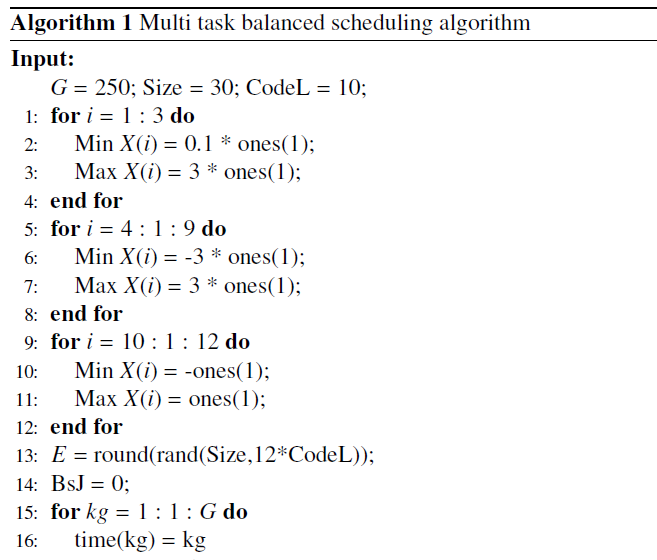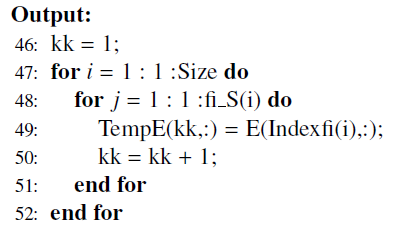7）例子7 \begin{algorithm}[!htb] \caption{Low latency video coding rate control algorithm} \label{alg1} \begin{algorithmic} \FOR{$n=1$to$N$} \STATE$G n=S B S_{n}$; \ENDFOR \STATE$G=\left\{G_{1}, G_{2}, \ldots G_{N}\right\}$\STATE$N=\{G, G, \ldots, G\}$\WHILE{The minimum value of$L$is less than$K$} \STATE Find out the subscripts of the two groups corresponding to the minimum value of$n^{*}, m^{*}$; \STATE$G_{m^{*}}$and$G_{n^{*}}$were removed from$G$; \STATE Merge$\left\{G_{n^{*}}, G_{m^{*}}\right\}$into$G$; \STATE Reset$L$to null; \FOR{$G_{n} \subset G$and$n>0$} \FOR{$G_{m} \subset G$and$m>n$} \STATE$L^{\prime} \leftarrow L\left(G_{n}, G_{m}\right)$;$m$\hspace{2pt}-\hspace{-2pt} -;$n$\hspace{2pt}-\hspace{-2pt} - \ENDFOR \ENDFOR \ENDWHILE \ENSURE group set$G$; \end{algorithmic} \end{algorithm} 样式：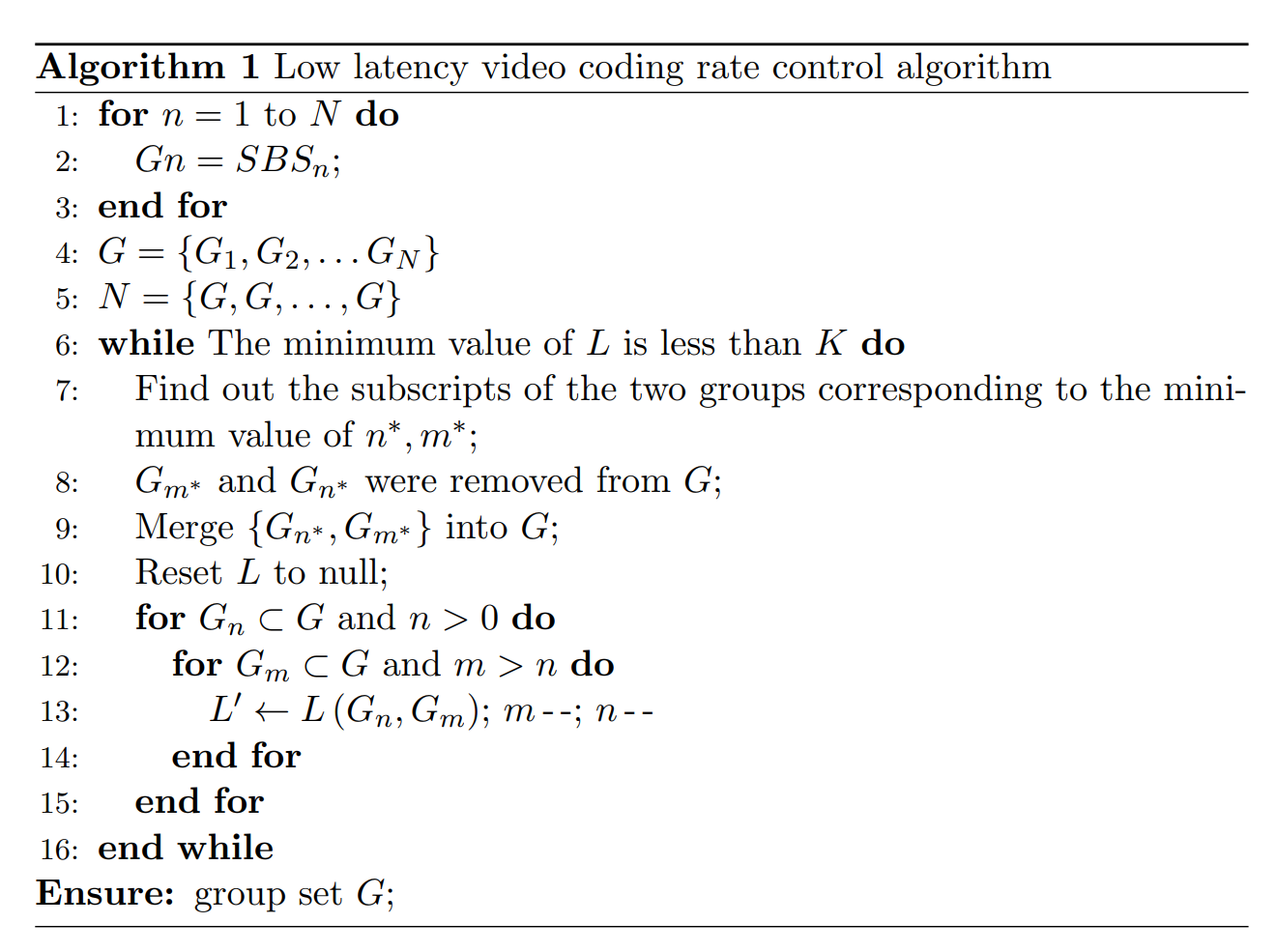展开全文• algorithm2e是一个非常优秀的宏包，可用于算法模板设计 实现代码为 更多详细介绍，可以参考文档 ... algorithm2e是一个非常优秀的宏包，可用于算法模板设计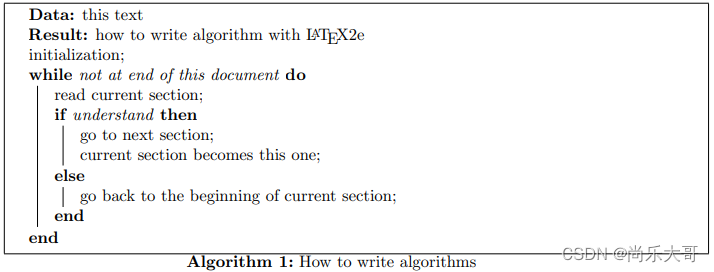实现代码为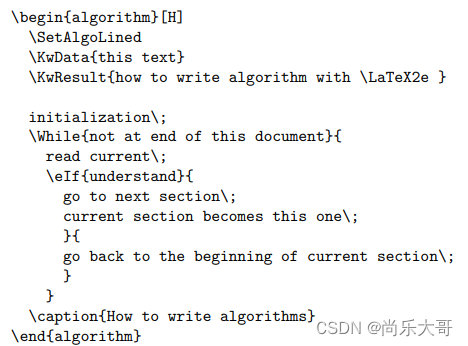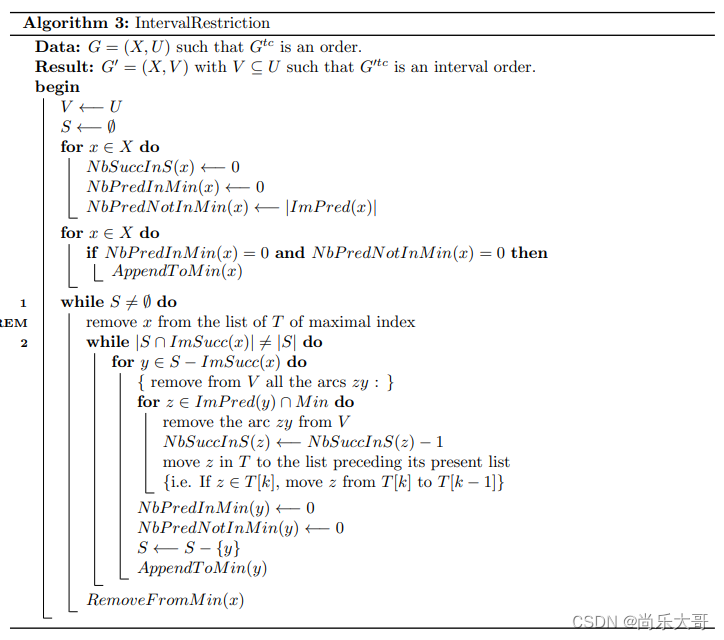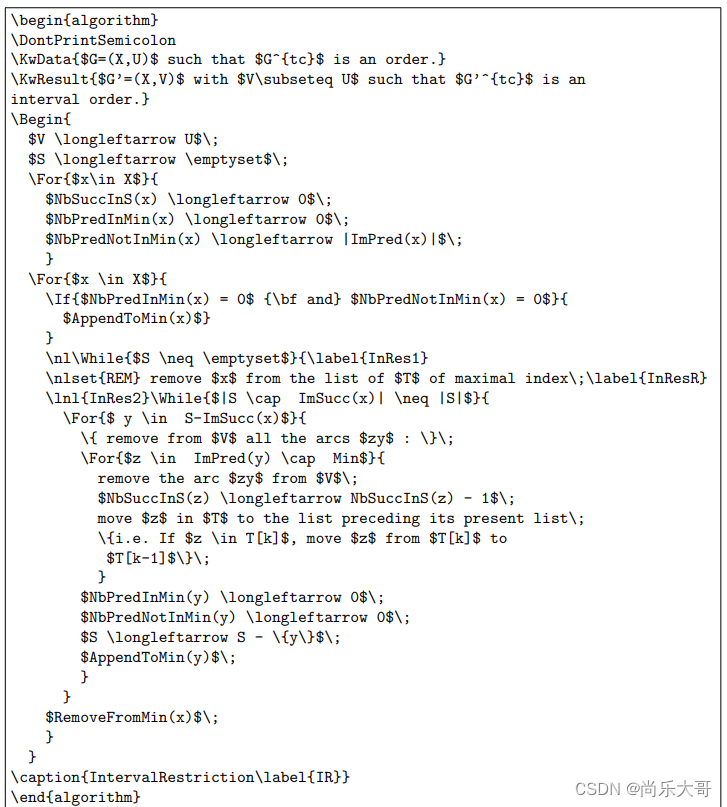更多详细介绍，可以参考文档 展开全文• ## 5. LaTeX算法伪代码基本格式排版 千次阅读 多人点赞 2021-11-20 00:34:12 1、算法排版初体验 LaTeX 的所有宏包文件都要写在 \documentclass 命令之后和\begin{document} 之前。在本文中，我们主要会用到如下的两个宏包： \usepackage{algorithmic} \usepackage{algorithm} 在论文写作的... # LaTeX 算法伪代码基本格式排版 ## 1、算法排版初体验 LaTeX 的所有宏包文件都要写在 \documentclass 命令之后和\begin{document} 之前。在本文中，我们主要会用到如下的两个宏包： \usepackage{algorithmic} \usepackage{algorithm}  在论文写作的过程中，对于计算机专业的学生，在论文中插入伪代码描述所用的算法还是比较常见的。这里我们先体验一下伪代码算法插入模板的效果，然后在后面详细讲解模板的中的每个细节。 我们比较通用的模板如下： \begin{algorithm}[!h] \caption{algorithm of SUM} \label{alg:AOA} \renewcommand{\algorithmicrequire}{\textbf{Input:}} \renewcommand{\algorithmicensure}{\textbf{Output:}} \begin{algorithmic} \REQUIRE$A$,$B$,$C$%%input \ENSURE EEEEE %%output \STATE AAAAA \WHILE{$A=B$} \STATE BBBBB \ENDWHILE \FOR{each$i \in [1,10]$} \IF {$C = 0$} \STATE CCCCC \ELSE \STATE DDDDD \ENDIF \ENDFOR \RETURN EEEEE \end{algorithmic} \end{algorithm}  上述模板的展示效果如下：## 2、算法排版基本用法 ### 1. 参数与语法说明 1、算法排版框架 \begin{algorithm}[!t] ... \end{algorithm}  整体大框架明确了伪代码或算法结构的开始（\begin）和结束（\end）的位置，而 algorithm 说明插入的内容是算法。[!h] 是算法的摆放格式：在当前位置(hear)，所有的格式和图片、表格相同。 2、标题与标签 \caption{Algor of ABC} \label{alg:AOA}  caption 是算法的标题。{ }中就是标题展示的具体内容，标题也会有编号，一般都是自动编号。label 是标签，标签主要是在引用的时候会用到。 说明：有时候，我们喜欢强调标签是属于算法标签，所以这里使用 alg:AOA，而不是直接使用 AOA 作为标签。 3、设置输入和输出 \renewcommand{\algorithmicrequire}{\textbf{Input:}} \renewcommand{\algorithmicensure}{\textbf{Output:}}  算法一般是需要输入和输出的，我们重新替换掉上面定义的两个命令： \algorithmicrequire \algorithmicensure所以修改之后，应该明白上面两行命令的含义了。我们使用的命令：\renewcommand{}{}，第一个大括号是修改前的命令，第二个大括号是修改后的命令。\textbf是命令文本的格式（加粗）。 如果这条语句适用于所有的算法，那么我们就可以把这条语句和引用包的命令放在一起：这就是全局设定的命令，所有用到该命令的算法都要遵守这个设定。在每个具体的算法中，我们就不需要使用这个命令了。但如果在算法中使用了这个命令，就会优先按照这个命令来。而其他没有使用这个命令，就是用前面全局设定的命令。 4、算法内容框架 \begin{algorithmic} \end{algorithmic}  这一对是算法内容的框架，表示算法的具体实现以伪代码的形式写在这里。 后面有个参数，参数中一般都写1，这个1表示具体算法内容前面的序号是每几行语句标一次序号，一般我们都是每一行算法语句都要，所以是1。5、输入输出 \REQUIRE$A$,$B$,$C$%%input \ENSURE EEEEE %%output  这一整块就是输入参数和输出参数。其中，\REQUIRE 命令表示输入，\ENSURE 命令表示输出。 6、算法内容 如果算法中不需要输入和输出这两条语句，那我们这两条语句就不用写在算法中,显示的效果就只有算法的内容了。\STATE AAAAA \WHILE{$A=B$} \STATE BBBBB \ENDWHILE \FOR{each$i \in [1,10]$} \IF {$C = 0$} \STATE CCCCC \ELSE \STATE DDDDD \ENDIF \ENDFOR \RETURN EEEEE  这一整块就是算法的内容了。每一条独立的语句，前面都要添加命令\STATE。除此之外，我们最常用的还有判断语句循环语句返回语句 ### 2. 算法的引用 算法的引用要用到的命令 /ref，如果要调用上面的算法，就可以这样调用： As show in algorithm \ref{alg:AOA}  命令大括号中的就是算法的标签，执行结果如下：### 3. 算法改名 有些时候，我们想用的不是 algorithm ，例如我们想写智能合约，就希望是下面这种样式：只需要在使用前添加一行：\floatname{algorithm}{Contract} \floatname{algorithm}{Contract} \begin{algorithm}[!h] \caption{Cont of ABC} \label{alg:AOA} \begin{algorithmic} \STATE AAAAA \end{algorithmic} \end{algorithm}  ## 3、算法常用语句 在算法的伪代码书写模式中有一些常用的语句，比如：条件分支、循环结构等。这些语法的使用要用到 algorithmic 宏包，即：\usepackage{algorithmic} ### 1. IF 条件判断语句 IF 条件判断的结构如下： \IF {条件} \STATE 语句1 \ELSE \STATE 语句2 \ENDIF  这个语句块的含义就是：如果条件成立，则执行语句1，否则执行语句2。如果我们不用考虑条件不成立，那么我们就可以省略 ELSE，直接写如下的语句块： \IF {条件} \STATE 语句1 \ENDIF  有两个以上的情况分支，就需要用到嵌套功能，即在一个判断语句中再写一个判断语句，例如： \IF {条件1} \STATE 语句1 \ELSE \IF{条件2} \STATE 语句2 \ELSE \STATE 语句3 \ENDIF \ENDIF  ### 2. FOR 循环语句 for循环语句主要用于那些具有明确循环次数和范围的循环语句，例如有如下的几种情况： \FOR{循环条件} \STATE 循环语句 \ENDFOR  这里以 1 到 100 以内所有整数之和为例： \STATE$Sum = 0$\FOR{each$i \in [1,100]$} \STATE$Sum = Sum + i$\ENDFOR  执行结果如下：### 3. while 循环语句 while循环主要用于那些循环次数不确定的，直到满足某个条件退出的，while循环格式如下： \WHILE{条件} \STATE 循环语句 \ENDWHILE  举个例子：计算 1 到 2021 的整数和。 \begin{algorithm}[!h] \caption{Sum} \label{alg:Sum} \begin{algorithmic} \STATE$s = 0$,$i = 1$\WHILE{$s<2021$} \STATE$s = s+i$\STATE$i++$\ENDWHILE \end{algorithmic} \end{algorithm}  上面实例的执行效果如下所示：展开全文• ## LaTex算法模板 千次阅读 2019-03-26 19:35:11 \Function {$Solovay\_Strassen$}{$n,times$}//Quick\_Mod为快速模幂算法,random产生随机数，Jacobi计算雅可比符号 \For{$i = 0 \to times$} \While{$Rand\_Num > 1 \&\& Rand\_Num $} \State$Rand\_...
• Latex-算法代码排版-algorithem2e 1.使用如下宏包 \usepackage[ruled]{algorithm2e} %带竖线 %\usepackage[ruled,vlined]{algorithm2e} %带竖线和折线 %\usepackage[linesnumbered,boxed]{algorithm2e} %方框格式 2...
• ## LaTeX算法排版例子

万次阅读 多人点赞 2013-03-26 20:31:27
\label{ code:fram:extract }%对此行的标记，方便在文中引用算法的某个步骤 \STATE Training ensemble of classifiers $E$ on $T_n \cup P_n$, with help of data in former batches; \label{code:fram:trainbase} ...
• ## LaTeX算法排版

万次阅读 2017-12-24 22:55:08
方式一　 需要包含的 \usepackage[noend]{algpseudocode} \usepackage{algorithmicx,algorithm} ...\caption{algorithm caption} %算法的名字 \hspace*{0.02in} {\bf In
• LaTeX宏包公式对齐算法格式排版 宏包   先导入宏包 \usepackage{algorithm} \usepackage{algorithmic} 公式对齐 等号对齐 代码 \begin{equation} \begin{aligned} \label{P} &\min_{c_{m,j}^{L},c_{i,j}^{V}...javascript
• 在网上搜索了一下，相关的中文期刊，《控制理论与应用》是有LaTex模板，奈何使用Ctex而不是用TexStudio。在TexStudio上使用总是出现乱码，或者不显示中文。 查阅相关资料后，了解到，大概造成上午问题原因主要有两...ieee论文
• ## LaTeX中文排版模板

千次阅读 2017-12-14 05:08:52
\documentclass[10pt,a4paper]{article}\usepackage{xcolor}\usepackage{titlesec}\usepackage{fontspec}\defaultfontfeatures{Mapping=tex-text}\usepackage{xunicode}\usepackage{xltxtra}\usepackage{polyglossia...
• LaTex试卷排版（1）目录正文 目录 关于选项的设置 关于图片的插入 正文 关于选项的设置 选项在同一行的代码，其中 {*{4}{@{}p{3.5cm}}} 表示4个选项，每个选项间隔距离3.5cm，这里的距离根据纸张大小设置。 \...
• 1、增加了算法伪代码的示例； 2、修复了\emph出现下划线的bug。 2021.07.23 V7版本 1、增加了cover页替换说明； 2、增加了粗体字体的代码； 3、增加了定理环境的代码。 2020.08.01 V6版本 1、修复了标题字体过大...
• 主要内容从以下链接转载： ...内容主要基于上述引用，但是上述引用在使用爱思唯尔模板的时候有些问题。 下面给出可用的代码。 设置环境： \usepackage{algorithm} \usepackage{algorithmic} \.
• LaTex 关于优化问题公式排版算法格式排版例子宏包LaTex公式书写公式对齐alignedsplit算法格式排版模拟退火算法 宏包 写算法别忘记导入算法的宏包： \usepackage{algorithm} \usepackage{algorithmic} LaTex公式...
• 这篇文章中总结了在排版过程中遇到的问题，希望可以大家可以注意。 1.公式 Mathtype复制公式可以直接转为LaTeX，需要在Mathtype中进行设置，旧的英文版Mathtype要在预设->剪切和复制预设->选择转为LaTeX；新...
• 一般情况下，如果有 LaTeX模板，就不用怎么考虑目录的格式，直接用命令 \tableofcontents 自动生成就好了。 但是有一些作业，想要用 LaTeX 排版，就要考虑老师给的格式，例如要自己重新设定各级标题的格式，对于...
• latex模板_适用于实验报告/课程设计与平时作业，专业性强，另附排版教程template
• 版权声明：本文为博主原创文章，遵循之前自己尝试用visio+mathtype画算法流程图，但是总是存...
• 公众号关注“视学算法”设为 “星标”，消息即可送达！来自 | GitHub 转自 | 机器之心参与 | Jamin、思、一鸣即使...这里整理了一些高赞开源项目 Readme、Gitignore 文档和论文的 LateX 模板。即使是初次研究的人也...
• 这里主要是对在 latex 中部分表格格式的方式做一个记录，以便之后会用到。 下面是用到的头文件，图片，表格，算法都写在一起了。可能部分读者需要自己用的，可以尝试将自己的表格格式改成下面表格中的某一种格式，...
• 1.Latex algorithmicx state语句跨行时对齐，作为一个整体的行，代码如下： \State \parbox[t]{\dimexpr\linewidth-\algorithmicindent}{...2.Latex algorithmicx实现算法排版时，在新的一行上添加注释且不占行号.
• 最近一直在写硕士毕业论文，在LaTeXStudio上发现了由@智博是小叮当编写的《大连海事大学硕博学位论文latex模板》，所以就开始尝试使用该模板来写毕业论文，有种前人种树后人乘凉的感觉。  模板下载地址  模板的...
• 修改某列 主要适用于某一列太宽或者太窄的情况 原来是： \begin{tabular}{|c|c|c|c|c|c|c|c|c|c|} 想把第一列改宽，修改为： \begin{tabular}{|p{1.7cm}<{\centering}|c|c|c|c|c|c|c|c|c|} ...
• 1、增加了算法伪代码的示例； 2、修复了\emph出现下划线的bug。 2021.7.23 V7版本 1、增加了cover页替换说明； 2、增加了粗体字体的代码； 3、增加了定理环境的代码。 2020.8.01 V6版本 1、修复了标题字体过大问题...
• 因为平时使用latex较少，本身对latex不是很熟悉，所以文本不会系统且全面的介绍如何使用latex制作一些精美的PPT、简历、报告、科技文章，也不会从头到尾教大家建议一套可用的latex模板。但是本文想尽力解决在拿到......